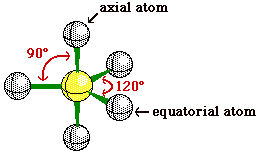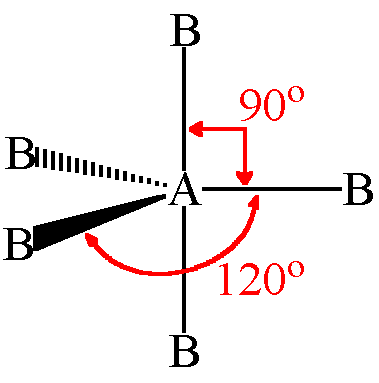﻿

Learn How Wiley is Aiding the Global Community in Response to COVID-19

﻿

# Valence Shell Electron Pair Repulsion Theory

The shape of various molecules was effectively explained by using the concept of hybridization and the non-visibility of the lone pair e.g. NH3, H2O etc. Now there was another problem which was the abnormal value of bond angle in molecules with irregular geometry e.g. in NH3 the pyramidal shape was explained but the abnormal bond angle of 1070 was shocking.

According the concept of hybridization, the orbitals must be equivalent in terms of special distribution around the central atom or should have an angle of 109028’ in NH3 molecule. Since the sp3 hybrid orbitals of N atom contains to different types of electron pairs i.e. the lone pairs and the bond pairs, it was clear that the behaviour of orbitals with lone pairs must be different from the ones carrying bond pairs.

Thus, the various characteristics of lone pairs and the bond pairs were studied especially the angular volume. It is quite obvious that angular volume is the prime factor that could affect the bond angles thus, angular volume or repulsion of an orbital containing lone and that containing bond pair was studied and following postulates of VSEPR theory were given:

• A lone pair is attracted by only one nucleus thus it is concentrated on one atom acquires a greater angular volume in contrast to a bond pair which is attracted by two nuclei and thus is stretched so it requires less angular volume.

• Thus due to the requirement of greater angular volume a lone pair repels more as compared to a bond pair due to acquire more space around the central atom. So, the order of the repulsions is L.P -L.P > L.P - B.P > B.P - B.P.

Occupation of more space around the central atom by a lone pair leads to lesser angular space available for the bond pairs around the central atom. Thus due to lesser available space or greater repulsion by the lone pair the bond angle decreases e.g. ÐHNH bond angle in NH3 molecule is reduced to 1070 due to greater repulsion of alone pair as compared to bond pairs.

Similarly the bond angle of H2O molecule equal to 104.50 and its lesser value than that of the bond angle of NH3 can be explained by using the order of repulsions i.e. L.P -L.P > L.P - B.P > B.P - B.P.

• If more than one structure is possible by placing lone pairs and the bond pairs at different positions, then the structure with minimum repulsions is the most stable and the correct one. For the comparison of the repulsions in various structures the number of various types of repulsions (L.P - L.P > L.P - B.P > B.P - B.P) at 900 are counted.
• The repulsions are counted in order of their strength starting from the strongest and the repulsions at 900 are counted only and all others are neglected e.g. in the molecule of SF4, the S atom is sp3d hybridized with trigonal bipyramidal geometry in the lone pair can be placed at two different positions i.e. the equatorial and the axial. Thus two different structures will be obtained as under:Among both the possible structures in SF4 as discussed above, the one having least repulsion will be more stable and thus correct which is found on the basis of the total number of repulsions counted in order of their decreasing strength at angle of 900 only as shown:

Thus the second structure having the lone pair at equatorial position is the more stable and the correct structure due to lesser repulsions. Also, the shape of the molecule can be deduced by hiding the lone pair and the bond angles can be explained by using the repulsion between electron pairs as under:

• A double bond requires greater angular volume too thus, behaves similar to a lone pair and thus always occupies equatorial position in case of trigonal bipyramidal geometry e.g. in the molecule of IOF3, the correct structure is as shown:

• Lastly the according to VSEPR theory, since the repulsion among two lone pairs is maximum and the repulsions at 900 are maximum; therefore, two lone pairs can never be present at 900 e.g. the species having any two lone pairs at 900 will never exist.

Read More in Wiley's Problem Book Inorganic Chemistry for JEE